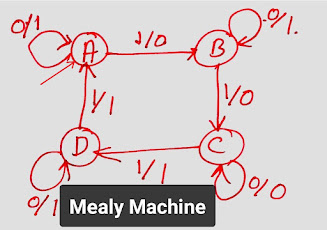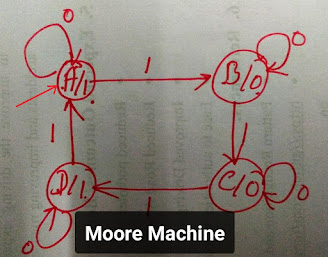# Diiference between Mealy and Moore machine

Mealy machine has 6 tuples: (Q, q0, ∑, O, δ, λ’)

1. Q : Finite set of states
• In diagram below Q = {A,B,C,D}
2. q0 : Initial state/ Starting state
• In diagram below A is initial state
3. ∑ : Input alphabet
• In diagram below input alphabets are {0,1}
4. O : Output alphabet
• In diagram below output alphabets are {0,1}
5. δ is transition function which maps Q×∑ → Q
6. ‘λ’ is the output function which maps Q×∑→ OMoore machine has 6 tuples: (Q, q0, ∑, O, δ, λ’)

1. Q : Finite set of states
• In diagram below Q = {A,B,C,D}
2. q0 : Initial state/ Starting state
• In diagram below A is initial state
3. ∑ : Input alphabet
• In diagram below input alphabets are {0,1}
4. O : Output alphabet
• In diagram below output alphabets are {0,1}
5. δ is transition function which maps Q×∑ → Q
6. ‘λ’ is the output function which maps Q → OMealy machine vs Moore machine

 Mealy machine Moore machine Output depends on present state as well as present input. Output depends on the present state. If input changes, output also changes If input changes, output does not changes. Compare to Moore less number of states are required. Because states do not depends on output. Compare to Mealy more number of states are required. Because states depends on number of output. Difficult to develop. Difficulty due to input affects output. Easy to develop. Output is placed on transition arrow. Output is placed with state.